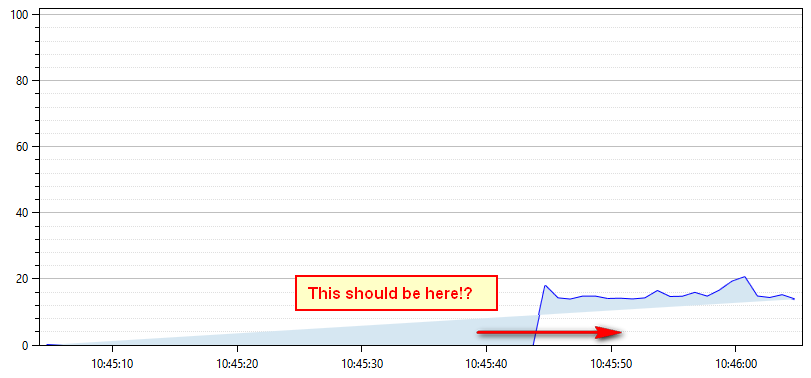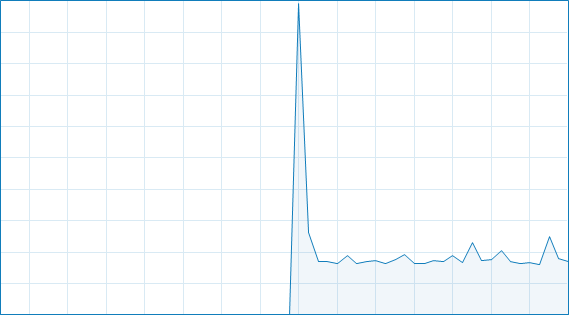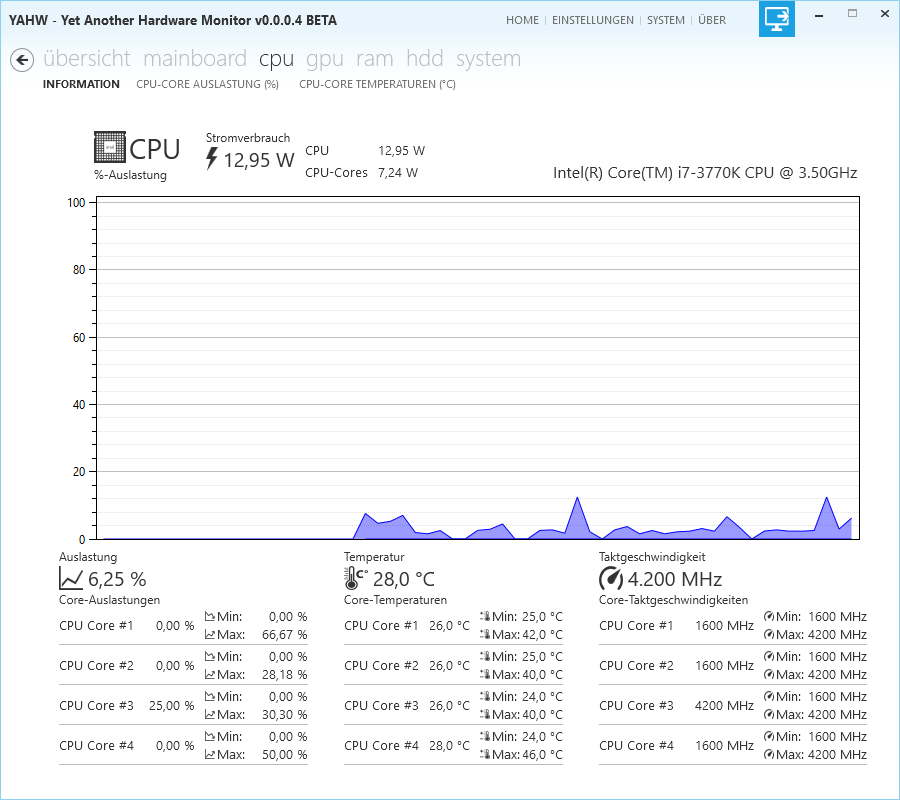+2
À l'étude

# Areaseries: Fill area below baseline (realtime)

Steve il y a 4 ans mis à jour par Oystein Bjorke il y a 4 ans
Hi,
currently I'm implementing a realtime chart and so far I have the following:But I want something like this:Any ideas how to achieve this? Here's the source code:

```/// <summary>
/// Setup Plot
/// </summary>
private void SetupPlot()
{
this.time = DateTime.Now;

this.Plot = new PlotModel();

{
IsZoomEnabled = false,
Maximum = 102,
Minimum=0,
MajorGridlineStyle = LineStyle.Solid,
MinorGridlineStyle = LineStyle.Dot,
Position = AxisPosition.Left
});

{
IsZoomEnabled = false,
Position = AxisPosition.Bottom,
});

var areaSeries = new AreaSeries()
{
StrokeThickness = 2,
LineStyle = OxyPlot.LineStyle.Solid,
Color = OxyColors.Blue,
Color2 = OxyColors.Transparent,
Fill = OxyColor.FromRgb(214, 231, 242),
DataFieldX2 = "X",
ConstantY2 = 0
};

// Fill series with initial values
for (int i = 0; i < 60; i++)
{
areaSeries.Points.Add(new DataPoint(DateTimeAxis.ToDouble(time.Subtract(new TimeSpan(0, 0, 60 - i))), 0));
}

}```
Update of the Plot (every second):
```void timer_Tick(object sender, EventArgs e)
{
var areaSeries = (AreaSeries)this.Plot.Series;

if (areaSeries.Points.Count > 60)
{
areaSeries.Points.RemoveAt(0);
}

// Update-Plot
double percentage = GetPercentage();

this.Plot.InvalidatePlot(true);
}```
Many Thanks!+2

Steve,

I've encountered the very problem and - even though the docs state that it should work using ConstantY2 - after skimming the source code I believe that this is not implemented at the moment. Anyway, you can easily work this around by using the Points2 collection

`areaSeries.Points.Add(DateTimeAxis.CreateDataPoint(this.time, percentage));areaSeries.Points2.Add(DateTimeAxis.CreateDataPoint(this.time, 0));`

This ain't really beautiful, but it works.+1

Hi P.k,

great it works now ;-) Thanks for the hint! Now that the chart looks like this:Perfect!

Best regards,

SteveÀ l'étude